# Geometrical-Optics Description of Step-Index and Graded-Index Optical Fibers

In its simplest form an optical fiber consists of a cylindrical core of silica glass surrounded by a cladding whose refractive index is lower than that of the core. Because of an abrupt index change at the core-cladding interface, such fibers are called step-index fibers.

In a different type of fiber, known as graded-index fiber, the refractive index decreases gradually inside the core.

This figure shows schematically the index profile and the cross section for the two kinds of fibers.Considerable insight in the guiding properties of optical fibers can be gained by using a ray picture based on geometrical optics. The geometrical-optics description, although appropriate, is valid when the core radius a is much larger than the light wavelength λ. When the two become comparable, it is necessary to use the wave-propagation theory.

#### 1. Step-Index Fibers

Consider the geometry of the following figure where a ray making an angle θi with the fiber axis is incident at the core center.Because of refraction at the fiber-air interface, the ray bends toward the normal. The angle of the refracted ray is given by:

n0sinθi = n1sinθr

where n1 and n0 are the refractive indices of the fiber core and air, respectively.

The refracted ray hits the core-cladding interface and is refracted again. However, refraction is possible only for an angle of incidence Φ such that sinΦ < n2/n1. For angles larger than a critical angle Φc, defined by:

sinΦc = n2/n1,

where n2 is the cladding index, the ray experiences total internal reflection at the core-cladding interface. Since such reflections occur throughout the fiber length, all rays with Φ > Φc remain confined to the fiber core. This is the basic mechanism behind light confinement in optical fibers.

We can use above two equations to find the maximum angle that the incident ray should make with the fiber axis to remain confined inside the core. Noting that θr = π/2 - Φc for such a ray and substituting it, we obtainIn analog with lenses, n0sinθi is known as the numerical aperture (NA) of the fiber. It represents the light-gathering capacity of an optical fiber. For n1 ≃ n2, the NA can be approximated by:where Δ is the fractional index change at the core-cladding interface. One would think that Δ should be made as large as possible in order to couple maximum light into the fiber. However, such fibers are not useful for the purpose of optical communications because of a phenomenon known as multipath dispersion, also called intermodal dispersion.

Multipath dispersion can be understood by referring to the last figure. Clearly, different rays travel along paths of different lengths. As a result, these rays disperse in time at the output end of the fiber even if they were coincident at the input end and traveled at the same speed inside the fiber. A short pulse (called impulse) would broaden considerably as a result of different path lengths. One can estimate the extent of the pulse broadening by simply considering the shortest and the longest ray paths. The shortest path occurs for θi = 0 and is just equal to the fiber length L. The longest path occurs for θi at the critical angle and has a length L/sinΦc. By taking the velocity of propagation v = c/n1, the time delay is given by: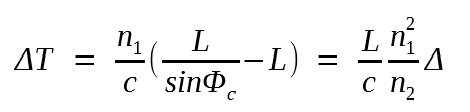The time delay between the two rays taking the shortest and longest paths is a measure of broadening experienced by an impulse launched at the fiber input.

We can relate ΔT to the information-carrying capacity of the fiber measured through the bit rate B. Although a precise relation between B and ΔT depends on many details such as the impulse shape, it is clear intuitively that ΔT should be less than the allocated bit slot (TB = 1/B). Thus an order-of-magnitude estimate of the bit rate is obtained from the condition BΔT < 1. We obtain: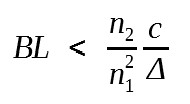This condition provides a rough estimate of a fundamental limitation of step-index fibers with a ≫ λ. ﻿As an illustration, consider an unclad glass fiber with n1 = 1.5 and n2 = 1. The bit rate-distance product of such a fiber is limited to quite small values such that BL < 0.4 (Mb/s)-km. Considerable improvement occurs for cladded fibers with a small index step. Most fibers for communication applications are designed with Δ < 0.01. As an example, BL < 100 (Mb/s)-km for Δ = 2 x 10-3. Such fibers can communicate data at a bit rate of 10 Mb/s over distances up to 10 km and may be suitable for some local area networks.

Two remarks are in order concerning the validity of the BL equation. First, it is obtained by considering only rays that pass through the fiber axis after each total internal reflection. Such rays are called meridional rays. In general, the fiber also supports the so-called skew rays which travel at angles oblique to the fiber axis. Skew rays scatter out of the core at bends and irregularities, and are not expected to contribute significantly to the last equation. Second, even the oblique meridional rays suffer higher losses than paraxial meridional rays because of scattering. The BL equation provides a conservative estimate, since all rays are treated equally. The effect of intermodal dispersion can be considerably reduced by using graded-index fibers. It can be altogether eliminated by using the single-mode fibers discussed later.

The refractive index of the core in graded-index fibers is not constant but decreases gradually from its maximum value n1 at the core center to its minimum value n2 at the core-cladding interface. Most graded-index fibers are designed to have a nearly quadratic decrease and are analyzed by using the so-called "α-profile", given by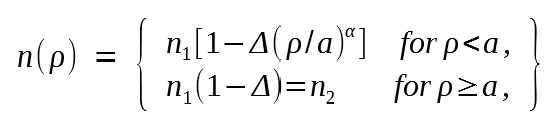where a is the core radius. The parameter α determines the index profile. A step-index profile is approached in the limit of large α. A parabolic-index fiber corresponds to α = 2.

It is easy to understand qualitatively why intermodal or multipath dispersion is reduced for graded-index fibers. The following figure shows schematically paths for three different rays. Similar to the case of step-index fibers, the path is longer for more oblique rays. However, the ray velocity changes along the path because of variations in the refractive index. More specifically, the ray propagating along the fiber axis takes the shortest path but travels most slowly as the index is the largest along this path. Oblique rays have a large path of their path in a medium of the lower refractive index where they travel faster. It is therefore possible for all rays to arrive together at the fiber output by a suitable choice of the refractive-index profile.Geometrical optics can be used to show that a parabolic-index profile leads to nondispersive pulse propagation within the paraxial approximation.

The trajectory of a paraxial ray is obtained by solvingwhere ρ is the radial distance of the ray from the axis. By using the index profile equation for ρ < a with α = 2, this equation reduces to an equation of harmonic oscillator and has the general solutionwhere p = (2Δ/a2)1/2 and ρ0 and ρ0' are the position and the direction of the input ray, respectively.

This equation shows that all rays recover their initial positions and directions at distances z = 2mπ/p, where m is an integer. Since the axial speed of all rays is the same, a parabolic-index fiber does not exhibit intermodal dispersion.

The above conclusion holds only within the paraxial and the geometrical-optics approximations, both of which must be relaxed for practical fibers. Intermodal dispersion in graded-index fibers has been studied extensively by using wave-propagation techniques. The quantity ΔT/L, where ΔT is the maximum multipath delay in a fiber of length L, is found to vary considerably with α. The following figure shows this variation for n1 = 1.5 and Δ = 0.01.The minimum dispersion occurs for α = 2(1-Δ) and depends on Δ as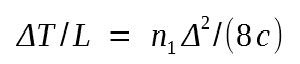The limiting bit rate-distance product is obtained by using the criterion ΔT < 1/B and is given by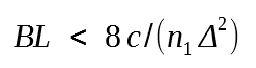The right scale in the above figure shows the BL product as a function of α. Graded-index fibers with a suitably optimized index profile can communicate data a bit rate of 100 Mb/s over distances up to 100 km. The BL product of such fibers [ ~ 10 (Gb/s)-km ] is improved by nearly three orders of magnitude compared with step-index fibers. Indeed, the first generation of lightwave systems used graded-index fibers. Further improvement is possible only using single-mode fibers. Geometrical optics cannot be used to study single-mode fibers. We will introduce wave propagation in optical fibers in the following tutorial.